# Bar Charts - Online Quiz

Following quiz provides Multiple Choice Questions (MCQs) related to Bar Charts. You will have to read all the given answers and click over the correct answer. If you are not sure about the answer then you can check the answer using Show Answer button. You can use Next Quiz button to check new set of questions in the quiz.Directions: Answer the questions given below using the graph below: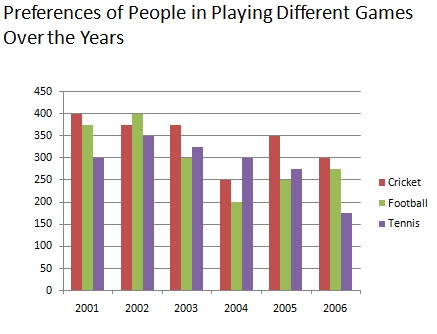Q 1 - How many people have preferred to play cricket considering all years together?

A - 2050

B - 2000

C - 1850

D - 1750

Explanation

```Required number = (400+375+375+250+350+300)= 2050 million.
```

Directions: Following bar diagrams depict the changes in student of a collage in four faculties from 2004- 05 to 2006-07. Study the diagram and answer the questions given below it: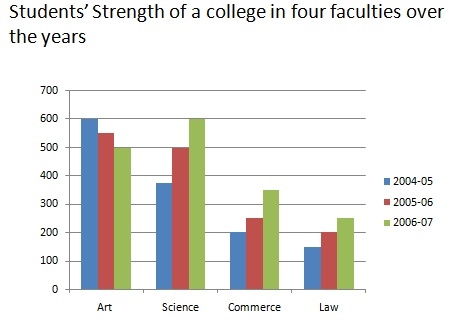Q 2 - In which faculty student strength was decreasing regularly?

A - Arts

B - Science

C - Commerce

D - Law

Explanation

```clearly , there was regular decrease in student strength in Arts faculty.
```

Directions: Study the given bar graph carefully and answer the questions given below: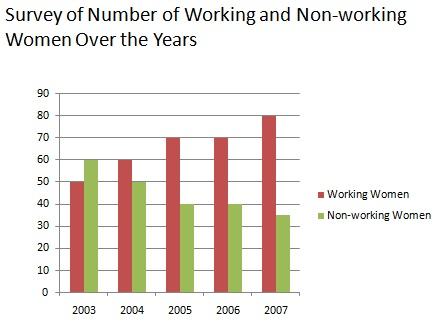Q 3 - Which of the following is the correct ratio of the number of women working in the year 2003 to the number of women working in the year 2007?

A - 3:4

B - 2:5

C - 1:3

D - 2:3

Explanation

```Required ratio  = 50 millions :75 millions = 2:3
```

Directions: Study the given bar graph carefully and answer the questions given below:Q 4 - In Year 2002, the number of people preferring to travel by rail in 2007 was how many millions fewer then the number of people preferring to travel by rail?

A - 70

B - 4

C - 75

D - 50

Explanation

```Required number = (350-275) millions = 75 millions.
```

Directions: Given below is a multiple bar diagram depicting the changes in student of a collage in four faculties from 2004- 05 to 2006-07. Study the diagram and answer the questions given below it.: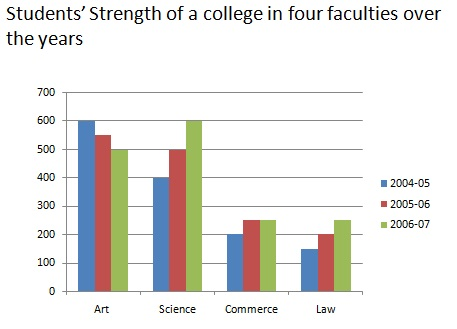Q 5 - What was the % of students in law faculty in 2006-07?

A - 18.5%

B - 15.6%

C - 16.7%

D - 14.8%

Explanation

```number of law students in 2006-07 =250
Total number of students = (500+600+250+250)= 1600
Required % = (250/1600*100)% = 15.6%
```

Directions: The following bar diagram shows the monthly expenditure on food ,clothing, education ,fuel ,house rent and miscellaneous. Study the diagram and answer the questions given below it: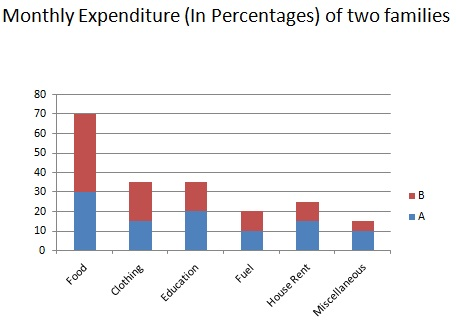Q 6 - If both the families have equal expenditure, which one spends more on education and miscellaneous together?

A - A

B - B

C - Both spend equally

D - None of above

Explanation

```Let the total expenditure of each be Rs.x.
Money spent by A on education and miscellaneous = (20+10)% of Rs.x = 30% of Rs .x
= Rs. (30/100*x) = Rs. 3x/10
Money spent by B on education and miscellaneous = (15+5)% of Rs.x = Rs. (20/100*x) = Rs.2x/10
Clearly , 3x/10 >  2x/10, A spends more.
```

Directions: The following bar diagram given below shows the result of B.S.C students of a collage for three years. Study the bar diagram and answer the questions given below: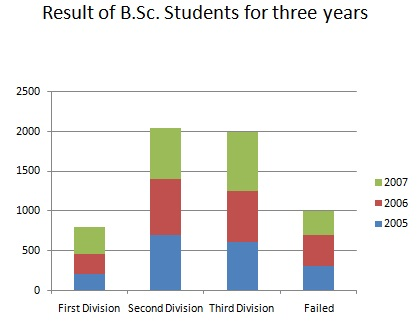Q 35 - Which is the following is the aggregate pass percentage during 3 years?

A - 51.66%

B - 82.9%

C - 80.4%

D - 77.6%

```Required % =  { (1500+1600+1750)/(1800+2000+2050)*100} = (4850/5850*100)%  = 82.9%